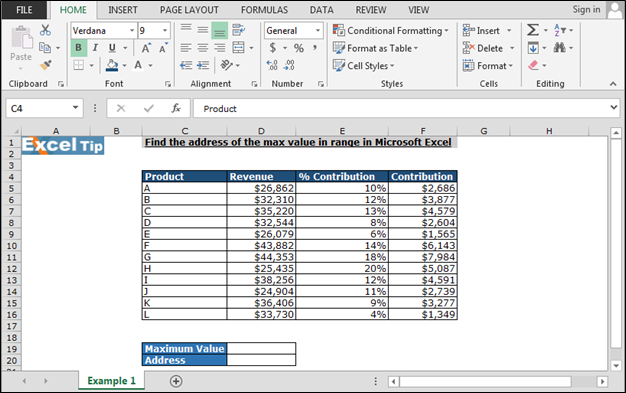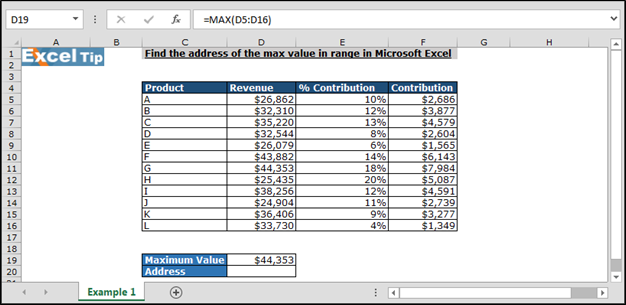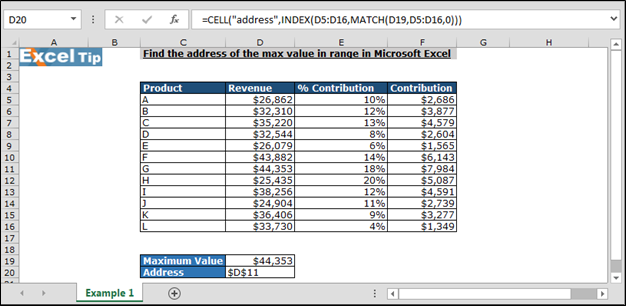# Find the address of the max value in range in Microsoft Excel

In this article, you will learn very tricky and useful Excel function to find out the address of the maximum value in a range. To find out the address of maximum valued cell, we are going to use combination of frequently used functions: - Max, Cell, Index and Match functions.

Now let’s see what all formulas will do to solve the problem

1. How Max function will help in this problem?

Max function will find out the maximum value.

1. What Cell function will do in this problem?

Cell function will help to get the cell address.

1. How Index and Match function will perform?

Index and Match function will look up the value and provide the cell to Cell function to return the cell Address.

Let’s take an example and then understand how it will work?

We have product revenue data in Excel in which we want to find out the Maximum value containing cell address. In data column, C contains product name, column D contains revenue, column E contains % of contribution, and column F contains contribution amount.First we will return maximum value, follow below steps:-

• Enter the formula in cell D19
• =MAX(D5:D16), Press Enter
• The function will return the maximum valueNow we will enter the formula to find out the address

• Enter the formula in cell D20
• The function will return address of the maximum value.In this way, we can find the address of the value in the range in Microsoft Excel by using the functions.If you liked our blog, please like us @ Facebook or you can follow us @ Twitter. Also, we are interested in knowing your views on our blogs and what type of blogs you liked the most or where we need to improve in the blogs for your better understanding.

1.